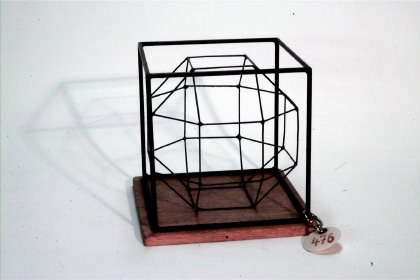Göttingen Collection of Mathematical Models and Instruments

# Cube with inscribed rhombicuboctahedron

### Model 476Category: B II 181

### Description

Cube with inscribed (6+8+12)-plane polygon with 24 vertices that is polar to the deltoidal icositetrahedron.

CubeThe cube is one of the five Platonic solids, see also model 702.

Inscribed (6+8+12)-plane polygon with 24 vertices The solid inscribed in the cube is a rhombicuboctahedron. The rhombicuboctahedron arises from the cube by truncating its vertices and truncating its edges afterwards. By truncating the vertices and edges eight new triangles and 12 squares are created and the previously existing squares are transformed to proportionally smaller squares. The rhombicuboctahedron is circumscribed by

8 triangles + 12 squares + 6 squares = 26 faces.

It has 4 · 6 = 24 vertices and 48 edges. At each vertice one triangles and three squares are meeting (3,4,4,4).

The rhombicuboctahedron is one of 13 Archimedean solids, see also 482.

Deltoidal icositetrahedron The rhombicuboctahedron is polar (dual) to the deltoidal icositetrahedron. To create this new solid a sphere is inscribed in the rhombicuboctahedron such that the sphere touches each of the faces in exactly one point. These points of contact create the vertices of the dual solid. By connecting these 26 vertices 24 deltoids are formed. These deltoids are the faces of the deltoidal icositetrahedron. The number of edges remains the same during the transformation into the dual solid, while the number of vertices and faces is exchanged.

There are 11 Archimedean solids in the collection.

 472 Truncated tetrahedron inscribed in a tetrahedron 473 Truncated octahedron inscribed in an octahedron 474485 Cuboctahedron 474 inscribed in an octahedron485 inscribed in a cube 475 Truncated cube inscribed in an octahedron 476 Rhombicuboctahedron inscribed in a cube 478 Truncated icosahedron inscribed in an icosahedron 479 truncated dodecahedron inscribed in a dodecahedron 480481 Icosidodecahedron 480 inscribed in a dodecahedron481 inscribed in an icosahedron 482 Rhombicosidodecahedron 483 Snub dodecahedron 484 Truncated cube inscribed in a cube

The truncated icosidodecahedron and the lost truncated cuboctahedron are not included in the collection.

Showcase of this model is Case number 21

### References

Heesch, H.. Comm.Math.Helv, 6, n=4, Casel 4a.

Hess, E.(1883). Kugelteilung, Teubner, mit Figuren, §35, fig. 19.